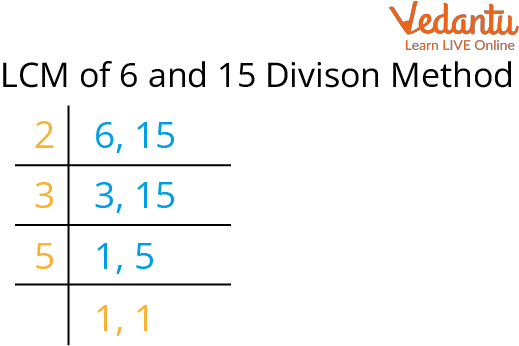Courses
Courses for Kids
Free study material
Free LIVE classes
More

# Questions on Least Common Multiple (LCM)LIVE
Join Vedantu’s FREE Mastercalss

## Introduction to LCM

In mathematics, LCM stands for a least common number. It can be used to pair two objects against each other. In easy language, we can say that the smallest repeated multiple between two or more numbers is found using the Least Common Multiple (LCM) method. We can calculate LCM by using three methods.Full Form of LCM

3. ### LCM using Division Method.

Now understand these methods with the help of examples.

## LCM by Listing Method

We can find out the common multiples of two or more numbers. Out of these repeated multiples, the LCM of two numbers can be calculated.

Let's take an example.

Q1. Find the LCM of numbers 4 and 5 by listing methods.

Ans: LCM of 4 and 5 by listing method:

4 is multiplied by: 4, 8, 12, 16, 20, 24, 28, 32, 36, 40, ..

and 5 is multiplied by: 5, 10, 15, 20, 25, 30, 35, 40, …

The first common multiple of 4 and 5 is 20

according to the least method the LCM of 4 and 5 is 20.

## LCM by Prime Factorisation Method

We can find out the prime factors of the numbers by using the prime factorization method. There are two steps to calculate the LCM using the prime factorization method.

Step 1: Put the numbers in the prime factored form

Step 2: The LCM of the given two numbers is the product of all the prime factors. (common factors will be included only once)

Understand this method using the example given below.

Q1. Find the least common multiple of 60 and 80

Ans: The prime factorization of 60 and 80 are:

60 = 2 × 2 × 3 × 5 = 2 × 2 × 3 × 5

80 = 2 × 2 × 2 × 5 × 2

LCM of 60 and 80 =2 × 2 × 3 × 2 × 5 × 2 = 240.

## LCM by Division Method

To calculate the LCM of two numbers using the division method we have to follow the steps given below:

• Step 1: Find a prime number that is a factor of at least one of the given numbers. Put this prime number to the left of the given numbers.

• Step 2: If the prime number in step 1 is a factor of the number, divide the number by the prime and write the quotient below. If the prime number in step 1 is not a factor of the number, write the number in the row below as it is. Continue the steps until only one is left in the last row.

## LCM Word Problems

1. Find the least common multiple (LCM) of 6 and 15 using the division method.

Ans:LCM by Division Method

LCM of 6 and 15 is 2 × 3 × 5 = 30.

2. What is the least common multiple of 980 and 9000 using the prime factorization method?

Ans: Prime factorization of 980 = 2 × 2 × 5 × 7 × 7 = 22 × 51 × 72 and

9000 = 2 × 2 × 2 × 3 × 3 × 5 × 5 × 5 = 23 × 32 × 53.

∴ LCM (980, 9000) = 23 × 32 × 53 × 72 = 441000.

# Q1. Find the LCM of 4, 6 and 12 by using the listing method.

Ans: 12

Q2. Find the LCM of 14 and 12.

Ans: 84

## Summary

### LCM is known as the lowest common number. LCM problems use three methods:

1. LCM using Listing Method

2. LCM using Prime Factorization

3. LCM using Division Method.

Last updated date: 26th Sep 2023
Total views: 122.4k
Views today: 4.22k

## FAQs on Questions on Least Common Multiple (LCM)

1. What are the methods to find LCM?

LCM can be calculated by using three different methods. These are as follows:

A. LCM using the Listing Method: the LCM of two given numbers can be calculated with the common multiple of two given numbers.

B. LCM using Prime Factorization: The LCM of the given two numbers is the product of all the prime factors. In this method, we have to keep in mind that common factors will be included only once.

C. LCM using Division Method: The division method is the most common, fastest, and easiest of these three methods. It can be used for both small and large numbers.

2. What is LCM and what is the most common and easy way to find the LCM? Give an example.

The smallest repeated multiple between two or more numbers was found using the Least Common Multiple (LCM) method. There are three methods to find the LCM.

Three ways to find the LCM are listing method, prime factorization, and division. The division method is the most common, fastest, and easiest of these three methods. It can be used for both small and large numbers. For example

Example: Find the LCM of 36 and 48 by using the division method.

Divide the numbers (36, 48) by their prime factors to get the LCM of 36 and 48 by using the division method. First, we divide the number by a common prime number and use the prime factor to calculate LCM.

 2 36 48 2 18 24 2 9 12 2 9 6 3 9 3 3 3 1 x 1 1

Hence, LCM of 36 and 48 is 144.